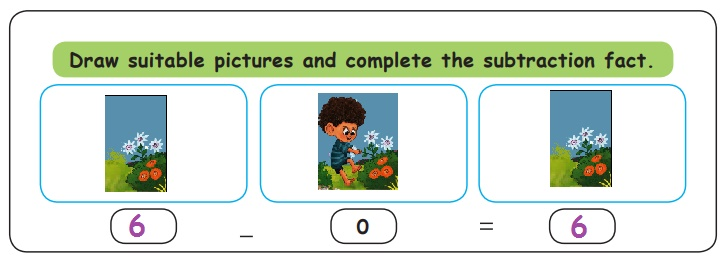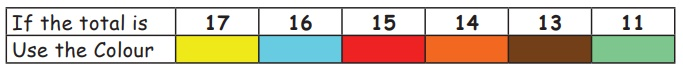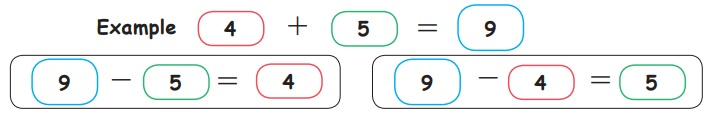Home | | Maths 1st Std | Subtracting Zero

# Subtracting Zero

Subtracting any number with the same number gives 0.

Subtracting Zero

Travel through

Care the nature.Number of flowers in the plant = 3

Number of flowers plucked by the girl = 0

Flowers left in the plant = 3

Learn

Subtraction using story.

Subtracting O from any number gives the same number.

3 – 0 = 3Practice

Complete the subtraction fact.Practice

Subtract2-0=2

4-0=4

5-0=5

9-0=9

1-0=1

6-0=6

8-0=8

Try this

Draw suitable pictures and complete the subtraction fact.Know more

Subtracting any number with the same number gives 0.

6 - 6 = 0

Try this

Say true or false. Why?

8-8=0

7-0=7

4-4=4

RecallFind the hidden picture in the above chart by colouring, using the following instructions.Know more

Creating two subtraction facts from the addition fact.

Example 4 + 5 = 9